The market demand for low cost and high performance wireless communication systems, including cellular phones, wireless local area networks (WLAN) and wireless personal area networks (WPAN), has attracted heavy investment and intensive research in the area of integrated RF transceiver design.1–9 Unlike a traditional discrete component radio design, where each building block, such as a low noise amplifier (LNA), filter or mixer, can be individually specified and verified, a fully integrated RF transceiver provides extremely limited access to the intermediate stages for testing, if any. Thus, the transceiver characterization often has to settle for only “pin-to-pin” measurements, namely, only the performance parameters of the total signal chain (such as the receiver signal path) can be evaluated. Test chips can be made to separately characterize each building block before they are finally integrated. However, making and characterizing test chips inevitably prolongs the development cycle and increases costs. Moreover, it is often difficult to accurately replicate the operating conditions, such as input/output impedance of the load/drive stages of the building blocks on the test chip, which mandates well-controlled measurement set-ups and laborious de-embedding procedures.It is therefore desirable to develop a test methodology for a fully integrated transceiver to obtain more than just “pin-to-pin” performance parameters. In this article, the third-order nonlinearity of a direct-conversion receiver (DCR) is evaluated with a set of well-designed tests. A DSI two-tone test is proposed to separate the nonlinearity of the RF section from the baseband section of the receiver. By combining the test results, the nonlinearity performance of the intermediate stages can be evaluated without having access to internal circuit nodes. Depending on the complexity of the receiver design (that is the number of stages), a complete mapping of the nonlinearity of all stages is also possible.

It is important to evaluate the linearity of the DCR for the following reasons:

• Modern receivers almost always need to accommodate a large range of input signal power level (for instance, the IEEE 802.11 WLAN receiver is required to reliably receive input signals from a few microvolts to tens of millivolts), which can only be fulfilled with a properly designed automatic gain control (AGC), so that none of the stages in the receive signal path would be saturated at a given input signal level. The knowledge of the input third-order intercept (IIP3) of each stage is therefore crucial in AGC gain table generation.

• The receiver is often required to reliably receive a weak desired signal in the presence of strong interfering signals residing in other channels. Due to the nonlinearity of the receiver, the interfering signals may intermodulate and generate beat signals in the desired channel, degrading the signal quality. The linearity of the receiver represents its capability in tolerating interference. The knowledge of the linearity performance of each stage in the receiver, or at least identifying the limiting stage of nonlinearity, is much desired in design optimization, such that optimal gain/filtering distribution and proper noise/linearity trade-off can be achieved.

When a received signal experiences nonlinearity, there are numerous intermodulation components and/or harmonics being generated. In practice, one only needs to focus on those impairments that fall in the desired channel, among which, the third-order intermodulation term (IM3) often becomes the dominant degrading factor. The second-order nonlinearity is also a critical performance parameter for a DCR10,11 but is beyond the scope of this article. It is worthy to note, however, that the technique described here can easily be extended to the evaluation of the second-order nonlinearity of a DCR.

THIRD-ORDER NONLINEARITY

In general, the static state behavior of any memory-less functional block (see Figure 1) can be represented approximately by a polynomial12

y(t) = f(x)

y(t) = a0 + a1x(t)

+ a2x2(t) + a3x3(t) + … (1)

As indicated before, only the third-order nonlinearity is considered here, thus

y(t) = a0 + a1x(t) + a3x3(t) (2)Fig. 1 Functional block f(x).

Input Referred Third-order Intercept Point (IIP3)12

The generation of a third-order intermodulation component (IM3) when two sinusoidal signals (Acosω1t, Acosω2t) are applied at the input of the functional block f(x) yields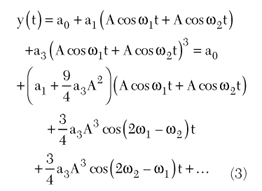For a small signal test, A can be small enough such thatThe input level for which the output components at ω1 and ω2 have the same amplitude as those at 2ω1–ω2 and 2ω2–ω1 is given byThis is commonly known as the input referred third-order intercept point (IIP3).

Input Referred Blocking 1 dB Gain Compression Point (IP1dB_b)

When one of the input signals (often known as the blocker) is significantly stronger than the other, that is

x(t) = A1 cosω1t + A2 cosω2t (5)

where A2 >> A1,

it can be shown thatIf a3 < 0, the weak signal A1cosω1t experiences progressively less gain as the other signal A2cosω2t gets stronger. The gain drops by 1 dB from its ideal value when the blocker amplitude reachesIt will be referred to as the input referred blocking 1 dB gain compression point (IP1dB_b), to differentiate it from the commonly known 1 dB gain compression point (IP1dB) to be defined in the next section.

Input Referred 1 dB Gain Compression Point (IP1dB)12

The condition when only one single tone sinusoid (Acosωt) is present at the input is now considered. Experiencing the nonlinearity, the input signal is transformed to an output given by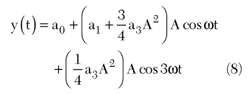Considering the coefficient of the fundamental term, the gain of the block f(x) is a decreasing function of the signal amplitude A if a3 < 0. When A reachesthe signal gain drops by 1 dB. The input signal power at which the gain drops by 1 dB is commonly known as the input referred 1 dB gain compression point (IP1dB).

Input Referred Third-order Harmonic Intercept Point (IIP3_h)

From Equation 8 another observation can be made: If the signal magnitude A is small, the third-order harmonic signal at the output has the same power level as if the input signal amplitude reaches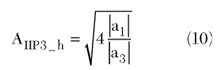It will be referred to as the input referred third-harmonic intercept point (IIP3_h).

So far four linearity parameters have been mathematically defined. There are, however, a couple of noteworthy remarks:

1. Although both IP1dB and IP1dB_b can be directly measured, the IIP3 and IIP3_h can only be extrapolated from a small signal measurement (more on this later) as they are defined under small signal assumptions.

2. From Equations 4, 7, 9 and 10, the following relations can be deduced:

IP1dB = IIP3 – 9.6 dB (11)

IP1dB_b = IIP3 – 12.6 dB (12)

IIP3_h = IIP3 + 5 dB (13)

However, these must be used with caution as the system in question must be reasonably close to the one represented by Equation 2.

Nonlinearity Measurement

The measurement of these nonlinearity parameters of a DCR is straightforward. Figure 2 shows a typical set-up that is sufficient for evaluating all four nonlinearity parameters. Two signal generators are used to facilitate the two-tone test. The device-under-test (DUT) is the DCR with a carrier frequency at ωLO, either built in the DUT or provided by a laboratory source. A spectrum analyzer with a frequency range of a few hundred megahertz is sufficient and, as in most applications, the desired signal frequency (ωBB) falls within a few tens of megahertz. All angular frequency parameters (ω1, ω2, ωLO, ωBB) are positive numbers in radians. As a commonly needed parameter for all the tests that follow, the gain (G) of the DCR is measured first.Fig. 2 Nonlinearity measurement set-up.

IIP3_h Measurement

Only one signal generator (ω1) is turned on and produces a sinusoid with the frequency set at ω1 = ωLO + ωBB. The baseband frequency ωBB must be low enough that its third-order harmonic (3ωBB) still falls within the passband of the DCR channel selection filter. Most importantly, the signal power at ω1 must be large enough to generate an observable third-order harmonic, but not too large to put any stage in the DCR into saturation (small signal assumption). The expected signal spectrum at the output is shown in Figure 3. Equation 8 shows that for every decibel increase of the signal power at ωBB, the third-order harmonic goes up by 3 dB. Thus, the output referred third-order harmonic intercept point (OIP3_h) isand its input referred value becomesFig. 3 IIP3_h measurement.

IP1dB Measurement

With the same measurement setting, the input signal power level at ω1 is increased, while monitoring the output signal at ωBB. An input-output transfer curve can be generated, as shown in Figure 4. The point at which the transfer curve deviates from the ideal small signal response by 1 dB is by definition the 1 dB gain compression point. The input referred is at IP1dB and the output referred is at OP1dB.Fig. 4 IP1dB measurement.

IIP3 Measurement

The IIP3 measurement is a small-signal two-tone test. Both signal generators are turned on and the frequency adjusted at ω1 = ωLO + ωBB1 for one and at ω2 = ωLO + ωBB2 for the other, with the same signal power levels. The expected output spectrum is shown in Figure 5, where in addition to the desired first-order components at ωBB1, and ωBB2 with power levels at P1, two IM3 components are generated at 2ωBB1 – ωBB2 and 2ωBB2 –ωBB1 with power levels at P3. The output referred third-order intercept point is thereforeand the input referred value becomesNote the similarity between Equations 15 and 17. Note also that the choice of the input signal power level is subject to similar constraints as in the IIP3_h measurement.Fig. 5 IIP3 measurement.

IP1dB_b Measurement

With the same settings as in the IIP3 measurement, when the signal power level at ω2 is increased, monitoring the output power level at ωBB1 will show a drop once the signal power at ω2 reaches a certain level. When the output signal at ωBB1 drops by 1 dB, the input signal power at ω2 is known as the blocking 1 dB gain compression point (IP1dB_b) and is shown in Figure 6.Fig. 6 IP1dB_b measurement.

Identifying the Limiting Stage in a DCR

Armed with these measurement techniques, the “pin-to-pin” linearity performance of a DCR can not only be evaluated, but crucial information for the intermediate stages in the signal path can also be obtained.

Without losing generality, consider the receiver design shown in Figure 7. The receiver consists of an LNA, a direct-conversion mixer (Mixer), a digitally controlled variable gain amplifier (A1), a low pass channel selection filter (LPF) and a last stage driver amplifier (A2). Each stage in the chain has its corresponding signal gain and its input-referred third-order intercept point (IIP3).Fig. 7 Block diagram of a direct-conversion receiver.

Dual-side Injection, Two-tone Test (DSI)

As described previously, the IIP3 measurement is traditionally performed with a two-tone test where the two input RF signal frequencies are either both higher than the local oscillator (LO) frequency (ω1 = ωLOBB1, ω2 = ωLO + ωBB2, low side injection) or both lower than the LO frequency (ω1 = ωLO – ωBB1, ω2 = ωLO – ωBB2, high side injection). In either case, two IM3 components (2ωBB1 – ωBB2 and 2ωBB2 – ωBB1) are generated and the contribution from the DCR RF section is indistinguishable from that of the BB section. Thus, by performing a traditional IIP3 measurement, one can only evaluate the DCR “pin-to-pin” linearity.

It is interesting to note that if a two-tone test is performed with dual-side injection (Figure 8), namely, one sinusoid is high side injection (ω1 = ωLO – ωBB1) and the other is low side injection (ω2 = ωLO + ωBB2), the nonlinearity contribution of the BB section can be separated from the RF section.Fig. 8 Input signal spectrum of a dual-side injection, two-tone test.

Let

ω1 = ωLO – ωBB1 (18)

ω2 = ωLO + ωBB2 (19)

At the output of the RF section (M), the expected frequencies are

m(ωLO – ωBB1)

+ n(ωLO + ωBB2) + kωLO (20)

where

m, n, k = 0, ±1, ±2, ±3, …

Considering the finite bandwidth at both the LNA output and the mixer output, one expects to observe:

First-order components:

ωBB1, ωBB2

Third-order components:

BB1 + ωBB2

--2ωBB2 + ωBB1

On the other hand, in the BB section, the first-order baseband signals (ωBB1, ωBB2) experiencing nonlinearity from point M to BBout, produce

BB1 + nωBB2 (21)

where

m, n = 0, ±1, ±2, ±3, …

Again, due to finite bandwidth, one expects to observe at BBout IM3 terms at (see Figure 9):

BB1 + ωBB2; 2ωBB2 + ωBB1

BB1 – ωBB2; 2ωBB2 – ωBB1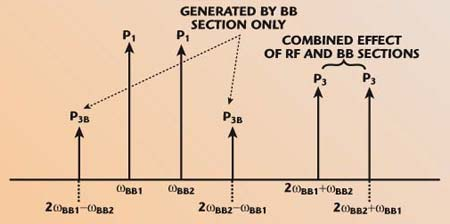Fig. 9 Output signal spectrum of a dual-side injection, two-tone test.

It is important to note that in the dual-side injection two-tone test, two IM3 terms (2ωBB1 – ωBB2; 2ωBB2 – ωBB1) can only be generated by the BB section. Therefore, the third-order intercept point of the BB section (IIP3BB, referred to the receiver input) can be calculated aswhere

The IIP3 of the receiver as a whole isBased on the nonlinearity analysis of cascaded stages, the IIP3 of the RF section can then be derived asSo far, without access to any intermediate nodes of the DCR, the third-order intercept points of the RF section, the BB section and the DCR as a whole have been measured in a single test.

Identifying the Limiting Stage

By comparing IIP3RF and IIP3BB, which section limits the linearity performance of the DCR can be identified. The RF section is limiting if IIP3BB is significantly better than IIP3RF, in which case the mixer stage usually dominates the nonlinearity. It is often not too difficult to verify by comparing the IIP3RF values for different LNA gain settings as implementing gain controls in the LNA is a common practice in modern receivers to accommodate the large range of the input signal power.Fig. 10 Third-order harmonic test with input signals outside of the LPF passband.

When it comes to the BB section, it is possible to take advantage of the filtering effect of the LPF to explore the linearity of each stage. As shown in Figure 10, two RF input signals at the same input power level produce first-order components with power levels at P11, P12, where their frequencies are so chosen such that

(1) P11–P12 = 1 dB

(2) ωLO + ωBB2 > ωLO + ωBB1 > ωLO

+ ωc

where

ωc = cut-off frequency of the LPF.

The magnitude response of the LPF indicates a roll off of –α dB/dec for signals outside of the passband. The third-order harmonic signals of ωBB1 and ωBB2 exhibit power levels at P31 and P32, respectively. The following two observations can be made. First, if the third-order harmonics are generated and only generated in the amplifier A1, they would experience the same filtering as their first-order counterparts and therefore follow the –α dB/dec line. Second, if the third-order harmonics are generated and only generated in the amplifier A2, the 1 dB power difference of the first-order tones at the output of the LPF results in a 3 dB difference in the power level of the third-order harmonics at the output of A2, assuming the amplifier bandwidth of A2 is sufficiently higher than 3ωBB2. In reality, the power level of the signal at 3ωBB2 would fall between the –α dB/dec line and the 3 dB line. The closer P32 is to the 3 dB or the (–α dB/dec) line, the more likely A2 or A1 is dominating the nonlinearity.

Another test is to measure the blocking 1 dB gain compression point (IP1dB_b) as a function of the blocker frequency (ωLO + ωBB2). Shown in Figure 11 is the IP1dB_b as a function of ωBB2, superimposed with the LPF transfer curve. An extreme case is when the IP1dB_b remains constant (dashed line) even when the blocker frequency ωBB2 falls outside of the LPF passband, indicating the amplifier A2 contributes a negligible nonlinearity.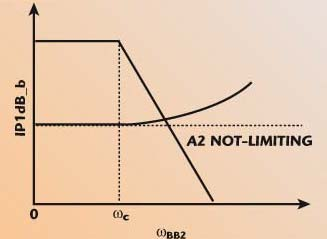Fig. 11 IP1dB_b as a function of the block-signal frequency.

Finally, if the BB section IIP3 (IIP3BB) follows the gain setting of A1 (see Figure 12), it is most likely that A1 is sufficiently linear and should not be the weak stage. Certainly this holds true only if the IIP3 of A1 does not change at different gain settings, which depends strongly on the actual implementation of the gain control.Fig. 12 IIP3BB as a function of the gain of A1.

Conclusion

This article reviewed the definitions and measurement techniques of the performance parameters representing the third-order nonlinearities of a receiver. Using a direct-conversion receiver (DCR) as a test case, a series of nonlinearity tests is designed to identify the limiting stage in the DCR. In particular, a dual-side injection, two-tone test (DSI) is proposed to separate the nonlinearity of the RF section from the one of the BB-section of a fully integrated DCR, without access to any intermediate circuit nodes.

Acknowledgments

The author would like to thank Prof. B. Razavi at UCLA for numerous instructive and insightful discussions. The author also wishes to thank K. Cai and D. Swaddell for experimenting with and verifying the techniques described.

References

1. P. Zhang, et al., “A 5 GHz Direct-conversion CMOS Transceiver for IEEE 802.11a WLANs,” IEEE International Solid-State Circuits Conference Digest, February 2003, pp. 354–355.
2. P. Zhang, et al., “A 5 GHz Direct-conversion CMOS Transceiver,” IEEE Journal of Solid-State Circuits, Vol. 38, No. 12, December 2003, pp. 2232–2238.
3. T.P. Liu, E. Westerwick, N. Rohani and R.H. Yan, “5 GHz CMOS Radio Transceiver Front-end Chipset,” IEEE International Solid-State Circuits Conference Digest, February 2000, pp. 320–321.
4. D. Su, et al., “A 5 GHz CMOS Transceiver for IEEE 802.11a Wireless LAN,” IEEE International Solid-State Circuits Conference Digest, February 2002, pp. 92–93.
5. H. Darabi, et al., “A 2.4 GHz CMOS Transceiver for Bluetooth,” IEEE International Solid-State Circuits Conference Digest, February 2001, pp. 200–201.
6. D.K. Shaeffer and T.H. Lee, “A 1.5 V 1.5 GHz CMOS Low Noise Amplifier,” IEEE VLSI Circuits Symposium Digest, June 1996, pp. 32–33.
7. A. Zolfaghari, A.Y. Chan and B. Razavi, “Stacked Inductors and 1-to-2 Transformers in CMOS Technology,” IEEE Custom Integrated Circuits Conference Proceedings, May 2000, pp. 345–348.
8. B. Razavi, “A 900 MHz CMOS Direct-conversion Receiver,” IEEE Symposium on VLSI Circuits Digest, June 1997, pp. 113–114.
9. B. Razavi, “A 900 MHz/1.8 GHz CMOS Transmitter for Dual-band Applications,” IEEE VLSI Circuits Symposium Digest, June 1998, pp. 128–131.
10. B. Razavi, “Design Considerations for Direct-conversion Receivers,” IEEE Transactions on Circuits and Systems II, Vol. 44, June 1997, pp. 428–435.
11. A.A. Abidi, “Direct-conversion Radio Transceivers for Digital Communications,” IEEE Journal of Solid-State Circuits, Vol. 30, December 1995, pp. 1399–1410.
12. B. Razavi, RF Microelectronics, Prentice Hall, Upper Saddle River, NJ, 1997.

Pengfei Zhang received his BS, MS and PhD degrees in electrical engineering from Tsinghua University, Beijing, China, in 1988, 1990 and 1994, respectively. From 1994 to 1996, he was a post-doctoral scientist in the electrical engineering department of the University of California at Los Angeles (UCLA), where he researched numerical simulation of SOI devices. From 1996 to 1999, he worked for Rockwell Semiconductors Inc., Newport Beach, CA, where he worked on advanced process technology development for a 56K-modem. From 1999 to 2000, he was employed with Fujitsu Microelectronics Inc., San Jose, CA. He worked on design methodology for signal integrity in mixed-signal ICs and RFIC design for wireless networking applications. Since 2000, he has been with RF Micro Devices Inc., where he has been managing the RFIC design group and RF systems group in the WLAN Division, working on transceiver chip development for multi-standard applications. His research interests include RF systems and integrated circuit design for wireless communications.